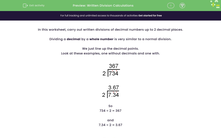# Solve Written Division Calculations

In this worksheet, students will carry out written divisions in cases where the answer has up to two decimal places.Key stage:  KS 2

Curriculum topic:   Number: Fractions, Decimals and Percentages

Curriculum subtopic:   Divide with Two Decimal Places

Difficulty level:#### Worksheet Overview

In this activity, we will carry out written divisions of decimal numbers up to 2 decimal places.

Dividing a decimal by a whole number is very similar to a normal division.

We just line up the decimal points.

Look at these examples, one without decimals and one with.So

734 ÷ 2 = 367

and

7.34 ÷ 2 = 3.67

Example

Use a written division to calculate:

8.45 ÷ 58 ÷ 5 = 1 r3

34 ÷ 5 = 6 r4

45 ÷ 5 = 9

How do you feel about some questions now? You can check back to this introduction at any point by clicking on the red help button.

### What is EdPlace?

We're your National Curriculum aligned online education content provider helping each child succeed in English, maths and science from year 1 to GCSE. With an EdPlace account you’ll be able to track and measure progress, helping each child achieve their best. We build confidence and attainment by personalising each child’s learning at a level that suits them.

Get started